# Difference between revisions of "Shortest path"

The shortest paths problem is one of the most fundamental problems in graph theory. Given a directed graph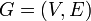$G = (V,E)$, possibly weighted, and a set of pairs of vertices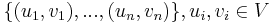$\{(u_1,v_1), ..., (u_n,v_n)\}, u_i, v_i \in V$, the problem is to compute, for each$i$, a simple path in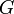$G$ from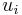$u_i$ to$v_i$ (a list of vertices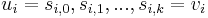$u_i = s_{i,0}, s_{i,1}, ..., s_{i,k} = v_i$ such that for all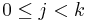$0 \leq j < k$,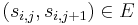$(s_{i,j},s_{i,j+1}) \in E$) such that no other simple path in$G$ from$u_i$ to$v_i$ has a lower total weight.

Shortest paths in undirected graphs can be computed by replacing each undirected edge with two arcs of the same weight, one going in each direction, to obtain a directed graph.

Theorem: In a graph with no cycles of negative weight, the shortest path is no shorter than the shortest simple path. (On the other hand, in a graph with a negative-weight cycle, lengths of paths may be unbounded below.)

Proof: We show that any path from$u$ to$v$ can be transformed into a simple path from$u$ to$v$ which is at least as short. Let the path be denoted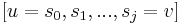$[u = s_0, s_1, ..., s_j = v]$. We proceed by induction on the number of pairs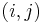$(i,j)$ (with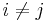$i \neq j$) such that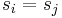$s_i = s_j$, which is countable. When there are zero such pairs, the path is already simple, and nothing needs to be done. Otherwise, we transform the path into another path with fewer such pairs but equal or lesser weight by removing the vertices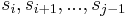$s_i, s_{i+1}, ..., s_{j-1}$ from the path. The weight of the path therefore decreases by an amount equal to the weight of the cycle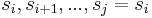$s_i, s_{i+1}, ..., s_j = s_i$, which is nonnegative.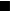$_\blacksquare$

Corollary: Assuming our graphs have no cycles of negative weight, the restriction that the shortest paths be simple is immaterial. Therefore, we will assume in the foregoing discussion that our graphs have no cycles of negative weight, for the problem of finding shortest simple paths in graphs containing negative-weight cycles is NP-complete.

Corollary: In a finite graph, a shortest path always exists. (To prove this we simply use the fact that the graph has a finite number of simple paths, and only simple paths need be considered. So one of them must be the shortest.)

## Variations

Three variations of the shortest path algorithm exist, and they are discussed in the following sections.

• In the single-pair shortest path problem, there is only one pair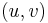$(u,v)$ in the problem set. In other words the shortest path is desired between a single pair of vertices.
• In the single-source shortest paths problem, the problem set is of the form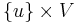$\{u\} \times V$. One vertex,$u$, is designated the source, and we wish to find the shortest paths from the source to all other vertices. (To solve the analogous single-destination shortest paths problem, we merely reverse the directions of all edges, which reduces it to single-source.)
• In the all-pairs shortest paths problem, the problem set is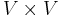$V \times V$; that is, we wish to know the shortest paths from every vertex to every other vertex.

## Approach

All the shortest paths algorithms discussed in this article have the same basic approach. At their core, they compute not the shortest paths themselves, but the distances. Using information computed in order to compute the distances, one can easily then reconstruct the paths themselves. They begin with the knowledge that the distance from any vertex to itself is zero, and they overestimate all other distances they need. (By this it is meant that they find a number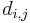$d_{i,j}$ for each pair$(i,j)$ under consideration such that the distance from$i$ to$j$ is less than or equal to$d_{i,j}$.) If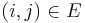$(i,j) \in E$, then the initial overestimate for the distance from$i$ to$j$ is the weight of the edge$(i,j)$; otherwise it is infinite. At some point, all overestimates will be refined, perhaps gradually, perhaps at once, so that once the algorithm has terminated, they are exactly the correct distances.

### Relaxation

There are theoretically many ways to refine overestimates but a specific way, known as relaxation, is used in all the algorithms discussed in this article. Relaxation can take place when three conditions are met:

1. The currently best overestimate for the distance from some vertex$i$ to some vertex$k$ is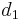$d_1$;
2. The currently best overestimate for the distance from$k$ to some vertex$j$ is$d_2$,
3. The currently best overestimate for the distance from$i$ to$j$ is greater than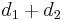$d_1+d_2$. (This includes the case in which it is infinite.)

Relaxation refines the best overestimate for the distance from$i$ to$j$ by setting it to$d_1+d_2$, which is better than its current value.

Theorem: When the distances from$u$ to all other vertices are all overestimated, but no relaxations are possible, then those distances are all known correctly. Contrapositively, if at there exists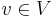$v \in V$ such that the distance from$u$ to$v$ is not correctly known, then relaxation must be possible somewhere in the graph.

Proof: By induction on the number of edges in some shortest path from$u$ to$v$ (which we can take to be simple). It is vacuously true when this is zero or one, because all paths of length zero or one were accounted for in the initial overestimates. Assume the path has at least two edges, and denote by$s$ the last vertex on the path before$v$. If the distance from$u$ to$s$ or from$s$ to$v$ is not known, then by the inductive hypothesis, we are done. Otherwise, notice that the path contains two subpaths, one from$u$ to$s$ and one from$s$ to$v$ (the latter is trivial as it consists of a single edge), and that each of these must itself be a shortest path, otherwise we could replace it with a shorter path to obtain a shorter path from$u$ to$v$, a contradiction. Now, as the distances from$u$ to$s$ and$s$ to$v$ are correctly known, and the correct distance from$u$ to$v$ is exactly the sum of the distances from$u$ to$s$ and$s$ to$v$ (as these values equal the weights of the aforementioned subpaths), and our overestimate of the distance from$u$ to$v$ is incorrect, it must be strictly greater than the sum of the distances from$u$ to$s$ and$s$ to$v$. Hence$(u,v)$ can be relaxed.$_\blacksquare$